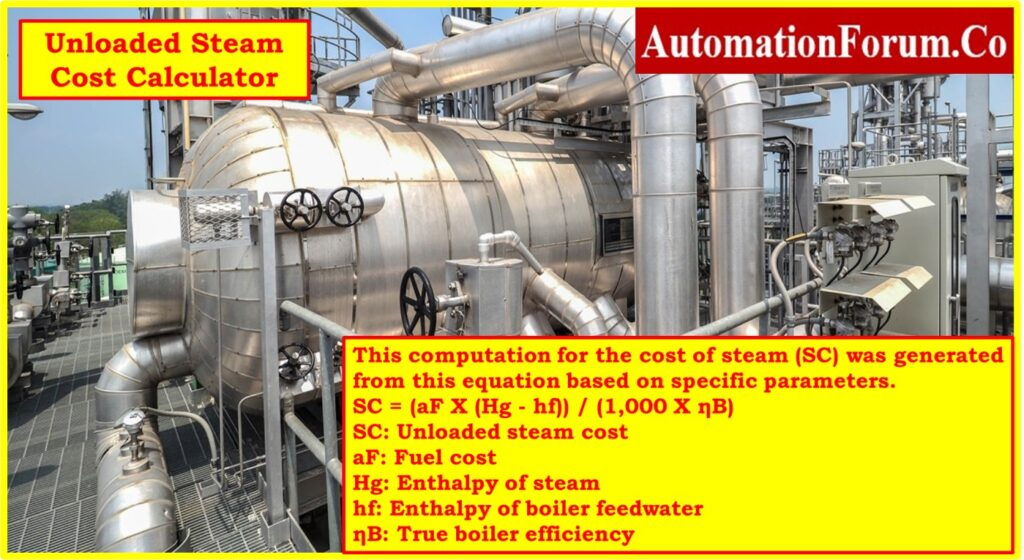## What is the unloaded steam cost?

The fundamental comparison between the amount of steam we produce and the price of the fuel we are buying is called the unloaded cost. Basic equation for determining the cost of fuel used by the boiler, the difference between the amount of steam it produces and the steam it produces from the feed water, and, of obviously, the boiler’s efficiency.

## Equation for Unloaded Steam Cost calculation

### Equation:

An equation for calculating the steam cost (SC) based on specific parameters.

SC = (aF * (Hg – hf)) / (1,000 • ηB)

### Variables:

• SC: Unloaded steam cost (cost per unit of steam, measured in units of 1,000 lbs. per hour)
• aF: Fuel cost (\$/Million Metric British Thermal Units, MMBtu)
• Hg: Enthalpy of steam (Btu/lb., energy content of steam)
• hf: Enthalpy of boiler feedwater (Btu/lb., energy content of feedwater)
• ηB: True boiler efficiency (decimal, per ASME PTC 4.1 method)
• 1,000: Conversion factor (cost measured in units of 1,000 lbs. per hour)

### Explanation:

The equation is used to estimate the cost of producing unloaded steam, which refers to steam generated by a boiler even when there is no active steam demand. This cost calculation accounts for the energy consumption required to generate steam that isn’t utilized for any specific purpose. The variables in the equation are crucial components in this estimation:

### aF (Fuel Cost)

This variable represents the cost of the fuel needed to generate steam. It is measured in dollars per Million Metric British Thermal Units (MMBtu). The fuel cost reflects the expense associated with the energy source utilized by the boiler.

### Hg (Enthalpy of Steam)

The enthalpy of the steam produced by the boiler is a measure of its energy content.It is measured in British Thermal Units per pound (Btu/lb). This value signifies the energy content of the steam when it exits the boiler.

### hf (Enthalpy of Feedwater)

This variable denotes the enthalpy of the boiler’s feedwater, which is the water that is eventually converted into steam. It is measured in Btu/lb. and signifies the energy content of the water prior to its transformation into steam.

### ηB (Boiler Efficiency)

Boiler efficiency is represented as a decimal and calculates the ratio of the boiler’s useful energy output (steam) to the energy input (fuel). It is based on the ASME PTC 4.1 method and indicates how effectively the boiler converts fuel energy into steam energy.

### 1,000

This factor is used to convert the result to units of cost per 1,000 lbs. of steam produced per hour.

## Example Calculation:

Let’s employ the given values for an example calculation:

• aF (Fuel Cost): \$8.50/MMBtu
• Hg (Enthalpy of Steam): 1200 Btu/lb
• hf (Enthalpy of Feedwater): 100 Btu/lb
• ηB (Boiler Efficiency): 0.85 (85%)
• 1,000 (Cost Unit): Cost measured in units of 1,000 lbs. per hour

To calculate the unloaded steam cost:

SC = (aF * (Hg – hf)) / (1,000 • ηB)

SC = (\$8.50/MMBtu * 1100 Btu/lb) / (1,000 * 0.85)

SC ≈ \$11.00/1,000 lbs.

The estimated unloaded steam cost is roughly \$11.00 per 1,000 lbs. of steam produced per hour.

This example serves as an illustration and doesn’t encompass all potential factors that may affect actual steam costs in intricate industrial settings. For precise cost calculations, it’s advisable to use specialized software or consult with experts knowledgeable about your specific steam generation system.